# 11-1100/Homework Assignment 3

Jump to: navigation, search

This assignment is due at class time on Tuesday, November 15, 2011.

### Solve the following questions

Problem 1. (Selick) Show that any group of order 56 has a normal Sylow-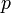$p$ subgroup, for some prime$p$ dividing 56.

Problem 2. (Qualifying exam, May 1997) Let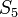$S_5$ act on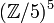$({\mathbb Z/5})^5$ by permuting the factors, and let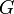$G$ be the semi-direct product of$S_5$ and$({\mathbb Z/5})^5$.

1. What is the order of$G$?
2. How many Sylow-5 subgroups does$G$ have? Write down one of them.

Problem 3. (Selick) Show that the group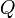$Q$ of unit quaternions (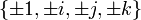$\{\pm 1, \pm i, \pm j, \pm k\}$, subject to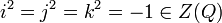$i^2=j^2=k^2=-1\in Z(Q)$ and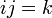$ij=k$) is not a semi-direct product of two of its proper subgroups.

Problem 4. (Qualifying exam, September 2008) Let$G$ be a finite group and$p$ be a prime. Show that if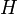$H$ is a$p$-subgroup of$G$, then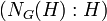$(N_G(H):H)$ is congruent to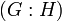$(G:H)$ mod$p$. You may wish to study the action of$H$ on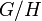$G/H$ by multiplication on the left.

Problem 5. (easy)

1. Prove that in any ring,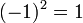$(-1)^2=1$.
2. Prove that even in a ring without a unit,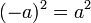$(-a)^2=a^2$.

(Feel free to do the second part first and then to substitute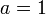$a=1$).

Problem 6.

1. (Qualifying exam, April 2009) Prove that a finite integral domain is a field.
2. (Qualifying exam, September 2008) Prove that in a finite commutative ring, every prime ideal is maximal.

Problem 7. (Dummit and Foote) A ring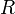$R$ is called a Boolean ring if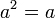$a^2=a$ for all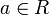$a\in R$.

1. Prove that every Boolean ring is commutative.
2. Prove that the only Boolean ring that is also an integral domain is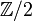${\mathbb Z}/2$.

Problem 8. (bonus) Let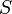$S$ be the ring of bounded sequences of real numbers with pointwise addition and multiplication, let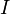$I$ be the ideal made of all sequences that are equal to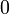$0$ except in at most finitely many places, and let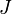$J$ be a maximal ideal in$S$ containing$I$.

1. Prove that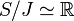$S/J\simeq{\mathbb R}$.
2. Denote by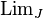$\operatorname{Lim}_J$ the projection of$S$ to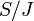$S/J$ composed with the identification of the latter with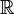${\mathbb R}$, so that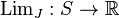$\operatorname{Lim}_J:S\to{\mathbb R}$. Prove that for any scalar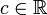$c\in{\mathbb R}$ and any bounded sequences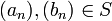$(a_n),(b_n)\in S$, we have that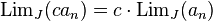$\operatorname{Lim}_J (ca_n)=c\cdot\operatorname{Lim}_J(a_n)$,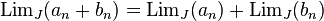$\operatorname{Lim}_J(a_n+b_n)=\operatorname{Lim}_J(a_n)+\operatorname{Lim}_J(b_n)$, and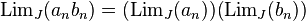$\operatorname{Lim}_J(a_nb_n)=(\operatorname{Lim}_J(a_n))(\operatorname{Lim}_J(b_n))$. (Easy, no bonuses for this part).
3. Prove that if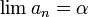$\lim a_n=\alpha$ in the ordinary sense of limits of sequences, then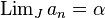$\operatorname{Lim}_Ja_n=\alpha$.
4. Is there a$J$ for which$\operatorname{Lim}_J$ is also translation invariant, namely such that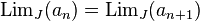$\operatorname{Lim}_J(a_n)=\operatorname{Lim}_J(a_{n+1})$? (Again, easy).

Warning. The right order for solving these questions is not necessarily the order in which they are presented.

Opinion. The sum total of all that is that using the axiom of choice you can construct things that are both too good to be true and not really useful anyway. Blame the axiom of choice, don't blame me.